Balbharti Maharashtra Board Class 5 Maths Solutions Chapter 1 Roman Numerals Problem Set 1 Textbook Exercise Important Questions and Answers.

## Maharashtra State Board Class 5 Maths Solutions Chapter 1 Roman Numerals Problem Set 1

Question 1.
Write all the numbers from 1 to 20 using Roman numerals.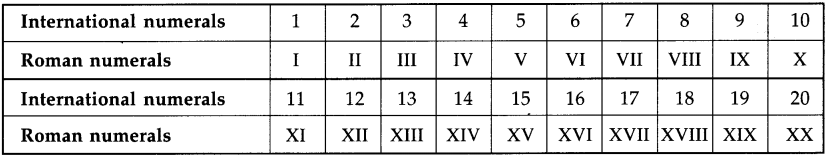Question 2.
Write the following numbers using international numerals.
(1) V
(2) VII
(3) X
(4) XIII
(5) XIV
(6) XVI
(7) XVIII
(8) IX
(1) 5
(2) 7
(3) 10
(4) 13
(5) 14
(6) 16
(7) 18
(8) 9

Question 3.
Fill in the empty boxes.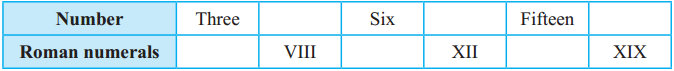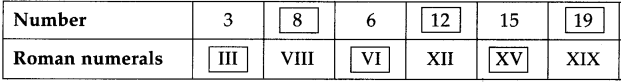Question 4.
Write the numbers using Roman numerals.
(1) 9
(2) 2
(3) 17
(4) 4
(5) 11
(6) 18
(1) IX
(2) II
(3) XVII
(4) IV
(5) XI
(6) XVIII

Question 5.
In the table below, each given number is written in international numerals and then again in Roman numerals. If it is written correctly in Roman numerals, put ‘/’ in the box under it. If not, put ‘X’ and correct it.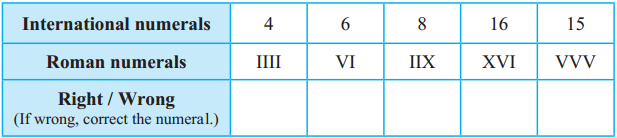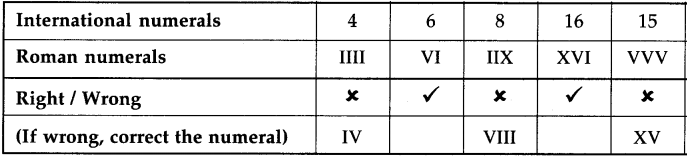Something more : L, C, D and M are also used as Roman numerals.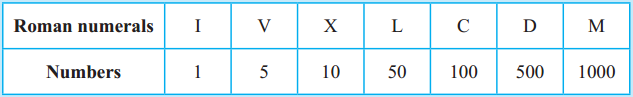Activity : Apart from clocks and watches, where else do we see Roman numerals?The decimal system of writing numbers
It is not easy to read or write numbers using Roman numerals. It also makes calculations difficult. You have learnt to write numbers using the ten digits, 0 to 9. In that system, the value of a digit depends upon its place in the number. This system of writing numbers is called the ‘decimal system’.

Mathematicians of ancient India invented the decimal system of writing numbers and began to use it. Later, this system was accepted in all parts of the world because it was simple and convenient.

Question 1.
Write all the numbers from 21 to 30 using roman numerals.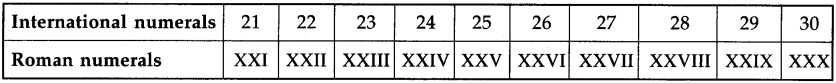Question 2.
Write the following numbers using international numerals.
(1) XXV
(2) XXIX.
(1) 25
(2) 29

Question 3.
Fill in the empty boxes: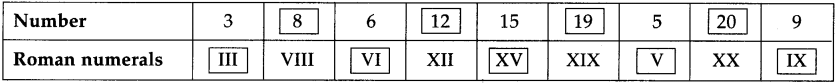Question 4.
Write the numbers using Roman Numerals:
(1) 1
(2) 21
(3) 10
(1) I
(2) XXI
(3) X

Question 5.
In the table below, each given number is written in international numerals and then again in Roman numerals. If it is written correctly in Roman numerals put ‘S’ in the box under it. if not, put ‘x’ and correct it.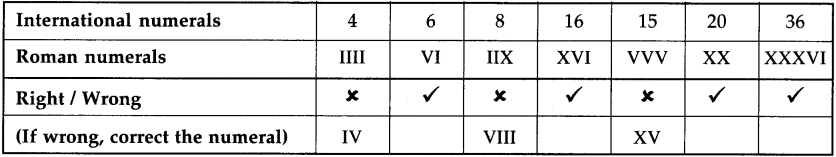Question 6.
Write all the numbers from 31 to 40 using Roman numerals.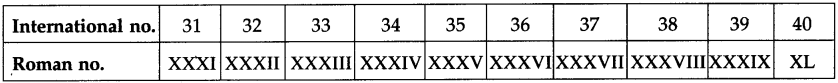Question 7.
Write the following numbers using international numerals.
(1) VI
(2) XI
(3) XIV
(4) XVII
(1) 6
(2) 11
(3) 14
(4) 17

Question 8.
Fill in the empty boxes: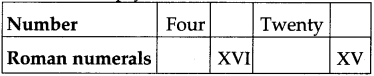Four = IV, 16 = XVI, Twenty = XX, XV =15

Question 9.
Write the numbers using Roman numerals in the given boxes.
(1) 3 [ ]
(2) 8 [ ]
(3) 13 [ ]
(4) 16 [ ]
(1) III
(2) VIII
(3) XIII
(4) XVI

Question 10.
State true or false of the following. If the statement is false then correct it in Roman numerals.
(1) 3 is written as IIV
(2) 14 is written as XIV
(3) 16 is written as XVI
(4) 19 is written as IXX
(1) False (III)
(2) True
(3) True
(4) False (XIX)Question 11.
(1) III + IV = [ ]
(2) IV + V = [ ]
(3) IX – X = [ ]
(4) VI + X = [ ]
(5) III + II = [ ]
(6) V + V = [ ]
(7) XXX + XX = [ ]
(8) L + L = [ ]
(1) VII
(2) IX
(3) XIX
(4) XVI
(5) V
(6) X
(7) L
(8) C

Question 12.
Match the columns: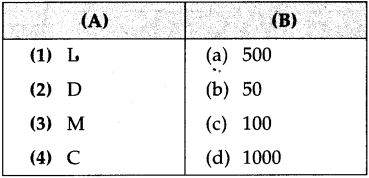(1 – b),
(2 – a),
(3 – d),
(4 – c)Question 13.
Circle the correct international numeral of the following:
(1) XXIV
(a) 14
(b) 20
(c) 24
(c) 24

(2) VIII
(a) 8
(b) 207
(c) 19
(a) 8

(3) XXIX
(a) 31
(b) 29
(c) 20
(b) 29

(4) XXVII
(a) 27
(b) 28
(c) 26
(a) 27Question 14.
Circle the correct Roman numeral of the following:
(1) 36
(a) XXVI
(b) XXXVI
(c) XXXXVI
(b) XXXVI

(2) 27
(a) XXXVI
(b) XXVII
(c) XXVI
(c) XXVII

(3) 18
(a) XVIII
(b) XVI
(c) XVII
(a) XVIII

(4) 605
(a)DCV
(b)CDV
(c) XXXXXV
(a) DCVQuestion 15.
Put >, < or = in the box.
(1) CVI [          ] CLX
(2) CLXIX [          ] CLXXI
(3) XLIX [          ] XXXIX
(4) MMXVI [          ] MMXXVI
(5) LXX VII [          ] LVIII
(6) MCMXL [          ] MCMXLV